# Factoring PolynomialsPage 3

#### WATCH ALL SLIDES

Positive factors of 30 Sum of Factors

1, 30 31

2, 15 17

Note, there are other factors, but once we find a pair that works, we do not have to continue searching.

So x2 + 13x + 30 = (x + 3)(x + 10).

Example

Slide 17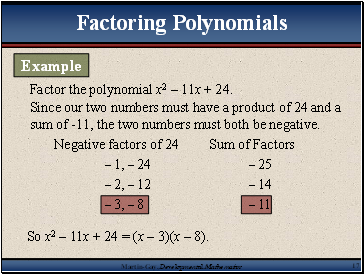Factor the polynomial x2 – 11x + 24.

Since our two numbers must have a product of 24 and a sum of -11, the two numbers must both be negative.

Negative factors of 24 Sum of Factors

– 1, – 24 – 25

– 2, – 12 – 14

So x2 – 11x + 24 = (x – 3)(x – 8).

Factoring Polynomials

Example

Slide 18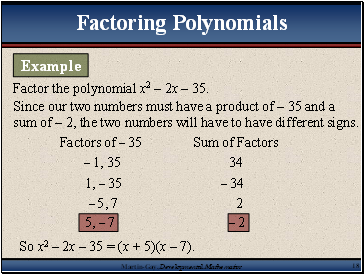Factor the polynomial x2 – 2x – 35.

Since our two numbers must have a product of – 35 and a sum of – 2, the two numbers will have to have different signs.

Factors of – 35 Sum of Factors

– 1, 35 34

1, – 35 – 34

– 5, 7 2

So x2 – 2x – 35 = (x + 5)(x – 7).

Factoring Polynomials

Example

Slide 19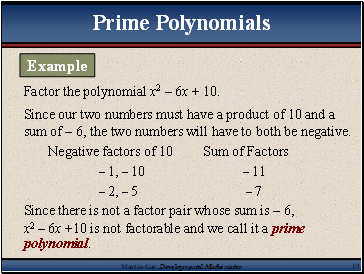## Prime Polynomials

Factor the polynomial x2 – 6x + 10.

Since our two numbers must have a product of 10 and a sum of – 6, the two numbers will have to both be negative.

Negative factors of 10 Sum of Factors

– 1, – 10 – 11

– 2, – 5 – 7

Since there is not a factor pair whose sum is – 6,

x2 – 6x +10 is not factorable and we call it a prime polynomial.

Example

Slide 20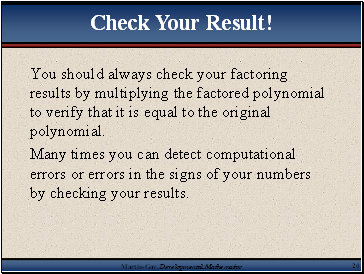You should always check your factoring results by multiplying the factored polynomial to verify that it is equal to the original polynomial.

Many times you can detect computational errors or errors in the signs of your numbers by checking your results.

Slide 21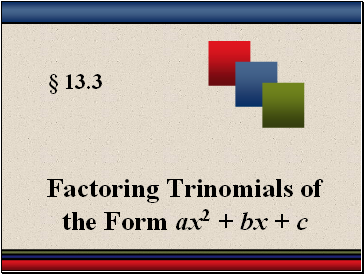§ 13.3

Factoring Trinomials of the Form ax2 + bx + c

Slide 22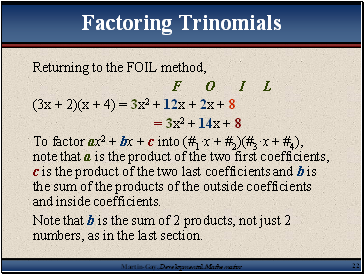## Factoring Trinomials

Returning to the FOIL method,

F O I L

(3x + 2)(x + 4) = 3x2 + 12x + 2x + 8

= 3x2 + 14x + 8

To factor ax2 + bx + c into (#1·x + #2)(#3·x + #4), note that a is the product of the two first coefficients, c is the product of the two last coefficients and b is the sum of the products of the outside coefficients and inside coefficients.

Note that b is the sum of 2 products, not just 2 numbers, as in the last section.

Slide 23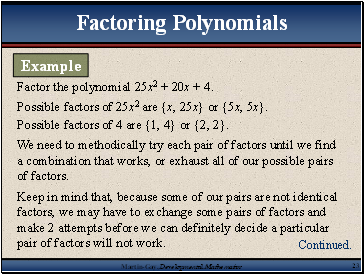## Factoring Polynomials

Factor the polynomial 25x2 + 20x + 4.

Possible factors of 25x2 are {x, 25x} or {5x, 5x}.

Possible factors of 4 are {1, 4} or {2, 2}.

We need to methodically try each pair of factors until we find a combination that works, or exhaust all of our possible pairs of factors.

Go to page:
1  2  3  4  5  6  7  8  9  10